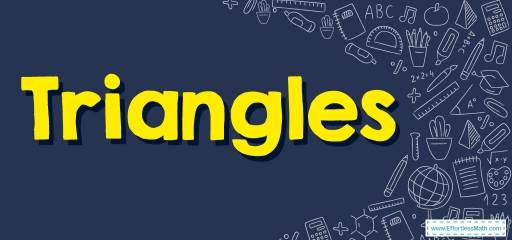# How to Solve Triangles Problems? (+FREE Worksheet!)

Learn how to solve mathematics problems related to triangles area and angles using common triangle formulas.## Step by step guide to solve Triangles

• In any triangle the sum of all angles is $$180$$ degrees.
• Area of a triangle $$= \color{blue}{\frac{1}{2 }(base \ × \ height)}$$### Triangles – Example 1:

What is the area of the following triangle?Solution:

Use the are formula: Area $$= \color{blue}{\frac{1}{2 }(base \ × \ height)}$$
base $$=10$$ and height $$=6$$
Area $$= \frac{1}{2} (10×6)=\frac{1}{2} (60)=30$$

### Triangles – Example 2:

What is the missing angle of the following triangle?

Solution:

All angles in a triangle sum up to $$180$$ degrees. Then: : $$45+60+x=180 → 105+x=180 → x=180-105=75$$

### Triangles – Example 3:

What is the area of the following triangle?

Solution:

Use the are formula: Area $$= \color{blue}{\frac{1}{2 }(base \ × \ height)}$$
base $$=12$$ and height $$=8$$
Area $$= \frac{1}{2} (12×8)= \frac{1}{2} (96)=48$$

### Triangles – Example 4:

What is the area of a triangle with base $$6$$ and height $$5$$?

Solution:

Use the are formula: Area $$= \color{blue}{\frac{1}{2 }(base \ × \ height)}$$
base $$=6$$ and height $$=5$$
Area $$= \frac{1}{2 } (5×6)= \frac{1}{2} (30)=15$$

## Exercises for Solving Triangles

### Find the measure of the unknown angle in each triangle.

1.2.3.1. $$\color{blue}{45^{\circ}}$$
2. $$\color{blue}{ 15^{\circ}}$$
3. $$\color{blue}{ 40^{\circ}}$$

### What people say about "How to Solve Triangles Problems? (+FREE Worksheet!) - Effortless Math: We Help Students Learn to LOVE Mathematics"?

No one replied yet.

X
30% OFF

Limited time only!

Save Over 30%

SAVE $5 It was$16.99 now it is \$11.99